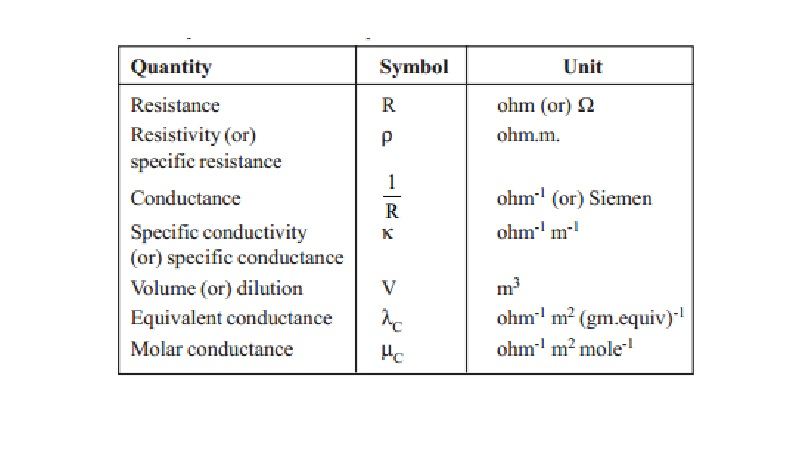Home | | Chemistry | Electrical Conductance Quantities

# Electrical Conductance QuantitiesThe S.I. unit of electrical current is ampere. The unit of quantity of electricity is coulomb. when one ampere of current is passed for one second, then the quantity of current passed is one coulomb. (i.e.,) Q = I x t Coulomb

ELECTRICAL CONDUCTANCE QUANTITIES

The S.I. unit of electrical current is ampere. The unit of quantity of electricity is coulomb. when one ampere of current is passed for one second, then the quantity of current passed is one coulomb.

(i.e.,) Q = I x t Coulomb

Ohm's law : This law can be stated as, at constant temperature, the strength of the current flowing through a conductor is directly proportional to the potential difference and inversely proportional to the resistance of the conductor.

Thus, I = V / R

V = IR

V = Volts, I = ampere, R = ohms

Specific resistance : The resistance 'R' ohms offered by the material of the conductor to the flow of current through it is directly proportional to its length (l) and inversely proportional to the area of cross section (a). Thus,

R a l/a   and

R =  ρ (l/a)

ρ  is called the specific resistance and it is resistance in ohms which one meter cube of material offers to the passage of electricitiy through it, unit of specific resistance is ohm-meter.

Specific conductance : The reciprocal of specific resistance is called as specific conductance (or) specific conductivity (k) [k is called 'kappa'].

k is defined as the conductance of one metre cube of an electrolyte solution

k = 1/ ρ  = 1/R . l/a

Unit of specific conductance is ohm-1 m-1 (or) mho.m-1

Since ohm-1 = mho

K = 1/ohm x m/m2  = ohm-1m-1

Also, 1 siemen = 1 mho. \ k is also expressed as S.m-1.

Conductance is the reciprocal of resistance 'R'.

Conductance = 1/R

( l/a ) is called as the cell constant (m-1) and is constant for a given conductance cell.

Thus specific conductance 'k' = cell constant x conductance  = Cell constant / Resistance

Equivalent conductance : Equivalent conductance (lC) is defined as the conductance of an electrolyte solution containing one gram equivalent of the electrolyte. It is equal to the product of specific conductance (k) of the solution and the volume (V) of the solution that contains one gram equivalent of the electrolyte.

(lC) = k x V

In general if an electrolyte solution contains N gram-equivalents in 1,000 cc of the solution the volume of the solution containing 1 gram equivalent  will be 1000/N x 10-6m3 (1 cc= 10-6m3 )

lC = k 10-3  / N mho.m2.(gm.equiv)-1

for 1 : 1 electrolyte normality N equals to molarity 'C'. Then

lC = k 10-3  / N mho.m2.(gm.equiv)-1

lC values depend on the type of the electrolyte, concentration of the solution and temperature.

Molar conductance : Molar conductance ' mC' is defined as the conductance of a solution containing one mole of the electrolyte dissolved in it.

= (k x 10-3 ) / C mho.m2.mole-1

where M is the molarity of the electrolyte solution.

For 1 : 1 electrolyte like NaCl, equivalent conductance is equal to molar conductance.

Study Material, Lecturing Notes, Assignment, Reference, Wiki description explanation, brief detail
11th 12th std standard Class Organic Inorganic Physical Chemistry Higher secondary school College Notes : Electrical Conductance Quantities |